# Class 6 Maths Basic Geometrical Ideas Important Questions

In this page we have Class 6 Maths Basic Geometrical Ideas Important Questions. Hope you like them and do not forget to like , social share and comment at the end of the page.
Topic of Circles,Triangles and Quadrilaterals is deleted from this chapter as per rationalized syllabus.So you may ignore those Questions
Question 1
Find the number of triangles in the below figure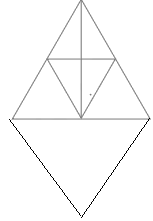Question 2
how many line segments are there? Name them.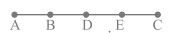Question 3
How many right angles are present in below figures And Name them also
a.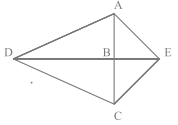Here AB ⊥ DE
b.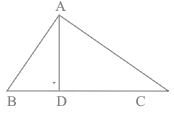Here AD ⊥ BC and ∠ BAC=900

Question 4
If the sum of two angles is equal to an obtuse angle, then which of the following is not possible?
(A) One obtuse angle and one acute angle.
(B) One right angle and one acute angle.
(C) Two acute angles.
(D) Two right angles.

Question 5
(a) A triangle is a _____________polygon
(b) The distance around the circle is called ________
(c) A ________ curve is one that does not cross itself.
(d) The join of any two non-adjacent vertices in a polygon is called _________
(a) Three-sided
(b) Circumference.
(c) Simple
(d) Diagonal

Question 6
Crossword Puzzle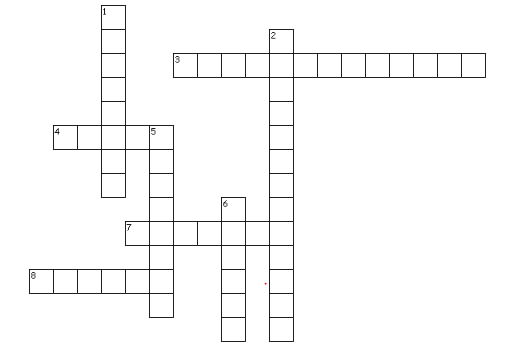Across
3. It is the distance around the circle
4. It is a line segment joining any two points on the circle.
7. it is a region in the interior of the circle enclosed by an arc and a chord.
8. it is the path of a point moving at the same distance from a fixed point.
Down
1. figures made entirely of line segments
2. It is a four-sided polygon
5. it is a chord passing through the centre of the circle.
6. it is the meeting point of a pair of sides

1. polygons
3. circumference
4. chord
5. diameter
6. vertex
7. segment
8. circle

• Notes and NCERT Solutions
• Assignments### Practice Question

Question 1 What is $\frac {1}{2} + \frac {3}{4}$ ?
A)$\frac {5}{4}$
B)$\frac {1}{4}$
C)$1$
D)$\frac {4}{5}$
Question 2 Pinhole camera produces an ?
A)An erect and small image
B)an Inverted and small image
C)An inverted and enlarged image
D)None of the above